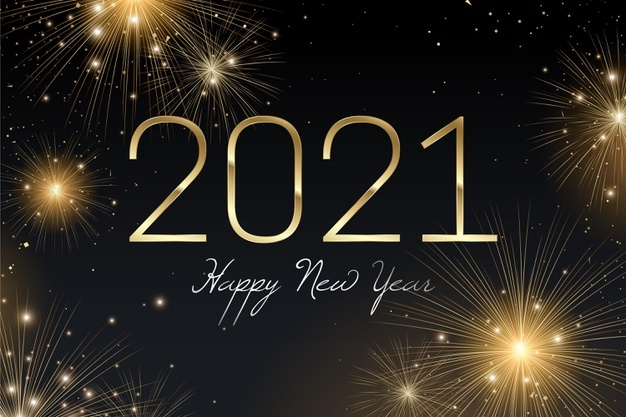# Upcoming Important Dates

Monday, January 4 - School resumes
Friday, January 8 - Virtual First Friday
Monday, January 18 - No school - Martin Luther King Jr. Day
Tuesday, January 19 - LSC meeting
Tuesday, January 26 - FON meeting

 table div table+table+table+table div table{width:100%;padding:0}table div table+table+table+table div table img{width:96.23%;padding:0;float:none}table div table+table+table+table div table td{width:100%;padding:0 1.88% 18px}/* styles */▪ Annual Appeal Update
▪ AP Exam Payment Info: Deadline to Pay is February 1, 2021
▪ LSC & FON Meetings
▪ Available Now: Custom NCP Brick With Your Personalized Message for Your Student
▪ Photo Submissions Requested for NCP Stampede!
▪ Order Your 2020-2021 NCP Yearbook
▪ Ways for Parents to Stay in Touch
 ▪ Annual Appeal Update
 ▪ AP Exam Payment Info: Deadline to Pay is February 1, 2021
 ▪ LSC & FON Meetings
 ▪ Available Now: Custom NCP Brick With Your Personalized Message for Your Student
 ▪ Photo Submissions Requested for NCP Stampede!
 ▪ Order Your 2020-2021 NCP Yearbook
 ▪ Ways for Parents to Stay in Touch
 table div table+table+table+table+table+table div table{width:100%;padding:0}table div table+table+table+table+table+table div table img{width:96.23%;padding:0;float:none}table div table+table+table+table+table+table div table td{width:100%;padding:0 1.88% 18px}/* styles */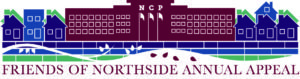# 2020-2021 Annual Appeal Campaign Continues

Friends of Northside received \$22,000 in donations in the past three weeks. Currently about 15% of the approximately 1,000 families at Northside have generously donated.

Below is another update on where our contribution levels stand compared to prior years at this time.

▪ 2020-2021 - \$102,000
▪ 2019-2020 - \$93,000
▪ 2018-2019 - \$150,000
▪ 2017-2018 - \$180,000
▪ 2016-2017 - \$183,000

If you are the parent of a sophomore, junior, or senior who has not contributed previously, we kindly ask if you would donate this year. For freshman parents new to Northside, many families choose to make donations in December or January towards the Annual Appeal.

Donations by check can be mailed to Northside. Donations by credit card or cash transfer via PayPal can be made by clicking on the donate button below.

Every dollar matters as they fund activities important to our students and which are not covered by the budget of Chicago Public Schools. Northside is annually ranked one of the top schools both in Illinois and the United States by U.S. News and World Report. Donations to Friends of Northside help fund activities to keep Northside the great school it is.

## Employer and Stock Donations

Please check with your employer about company matching donations. It can often double your support to Northside. Contact Friends of Northside at info@friendsofnorthside.org with any questions.

Friends of Northside also graciously accepts donations of stock, which we convert to cash upon receipt. Please contact us at info@friendsofnorthside.org. FON can easily provide information related to Wintrust Bank if you would like to donate stock to FON in lieu of cash

 table div table+table+table+table+table+table+table+table+table+table+table div table{width:100%;padding:0}table div table+table+table+table+table+table+table+table+table+table+table div table img{width:96.23%;padding:0;float:none}table div table+table+table+table+table+table+table+table+table+table+table div table td{width:100%;padding:0 1.88% 18px}/* styles */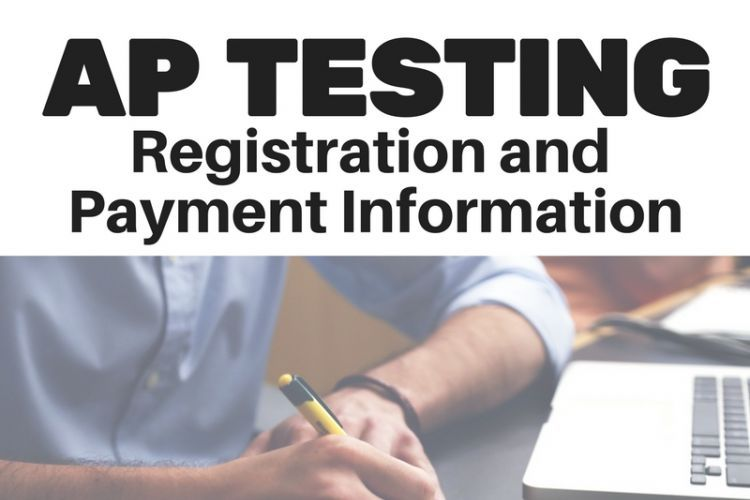# AP Exam Payment Info: Deadline to Pay is February 1, 2021

Students currently enrolled in AP courses may now pay for their exams. AP exam fees have been added to student accounts in ASPEN. The amount shown is the exact amount owed for those exams specifically. Please follow the instructions below to make payments by Monday, February 1, 2021.

Students currently enrolled in AP courses may now pay for their exams. AP exam fees have been added to student accounts in ASPEN. The amount shown is the exact amount/total owed for those exams specifically.

## To Pay:

1. Click on Family.
2. Click on Transactions.
3. Click on Fees. There you should see the AP Exam(s) for your student.
4. Click on Pay Online. This will take you to the e-Pay website.
5. Select AP Classes/exam and the correct cost based on the total amount owed for AP exams in your ASPEN account.

At this time, we are not able to take cash or physical check payments. This is a CPS policy for this year.

The NEW AP PAYMENT deadline is Monday, February 1.
*Fees have been waived for students who have an approved fee waiver for the 2020-21 school year.

 table div table+table+table+table+table+table+table+table+table+table+table+table+table+table div table{width:100%;padding:0}table div table+table+table+table+table+table+table+table+table+table+table+table+table+table div table img{width:96.23%;padding:0;float:none}table div table+table+table+table+table+table+table+table+table+table+table+table+table+table div table td{width:100%;padding:0 1.88% 18px}/* styles */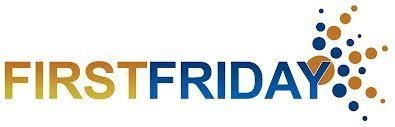# First Friday Virtual Gathering

Friday, January 8
9:00 am Zoom gathering

Each month, NCP parents and guardians are invited to get together for a monthly coffee gathering. This month, join fellow NCP parents online. Stay for as long as your schedule allows.

## Join Zoom meeting

▪ Meeting ID: 848 7451 7349
▪ Passcode: 336446

 table div table+table+table+table+table+table+table+table+table+table+table+table+table+table+table+table div table{width:100%;padding:0}table div table+table+table+table+table+table+table+table+table+table+table+table+table+table+table+table div table img{width:96.23%;padding:0;float:none}table div table+table+table+table+table+table+table+table+table+table+table+table+table+table+table+table div table td{width:100%;padding:0 1.88% 18px}/* styles */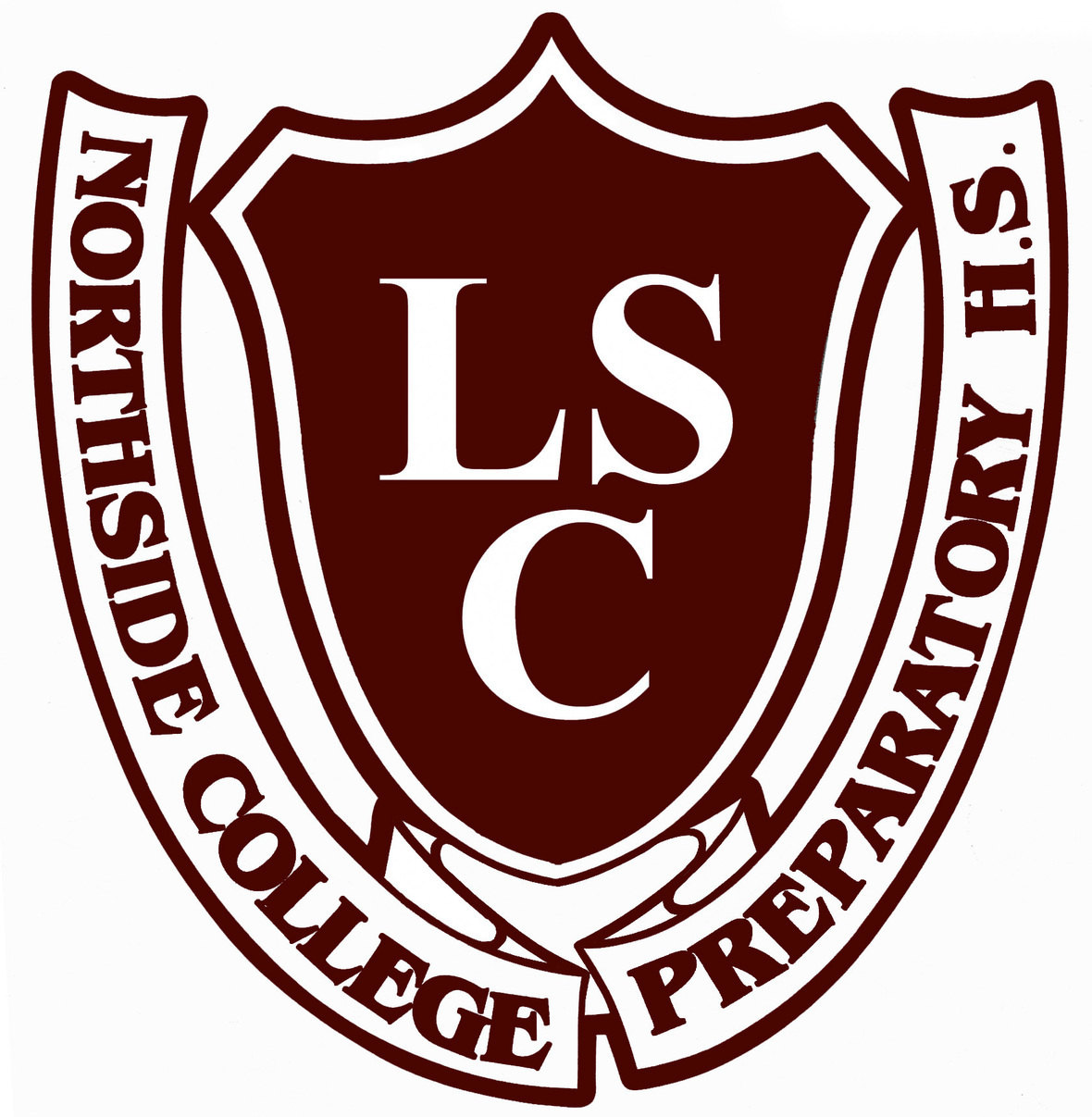# LSC Meeting

Tuesday, January 19
6:30 pm
Zoom Meeting

Click [HERE] for the agenda.

Meeting ID: 819 7253 0679
Passcode: 061702
One tap mobile:
+13126266799,,81972530679# US (Chicago)

 table div table+table+table+table+table+table+table+table+table+table+table+table+table+table+table+table+table+table div table{width:100%;padding:0}table div table+table+table+table+table+table+table+table+table+table+table+table+table+table+table+table+table+table div table img{width:96.23%;padding:0;float:none}table div table+table+table+table+table+table+table+table+table+table+table+table+table+table+table+table+table+table div table td{width:100%;padding:0 1.88% 18px}/* styles */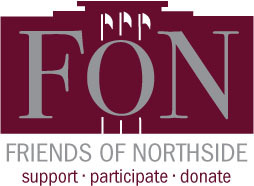# Friends of Northside

Tuesday, January 26
7:00 pm

Please join Friends of Northside for their upcoming meeting.

Friends of Northside (FON) is the “one-stop-shop” for NCP families to get involved. FON facilitates communication between parents and the school, organizes parent volunteers, plans and sponsors social/educational events, and raises much-needed funds to bridge the gap due to CPS budget cuts. FON supports the school’s mission to maintain and enhance the learning experience for all students of Northside College Prep High School.

To find out more about Friends of Northside, please visit their website HERE.

 table div table+table+table+table+table+table+table+table+table+table+table+table+table+table+table+table+table+table+table+table div table{width:100%;padding:0}table div table+table+table+table+table+table+table+table+table+table+table+table+table+table+table+table+table+table+table+table div table img{width:96.23%;padding:0;float:none}table div table+table+table+table+table+table+table+table+table+table+table+table+table+table+table+table+table+table+table+table div table td{width:100%;padding:0 1.88% 18px}/* styles */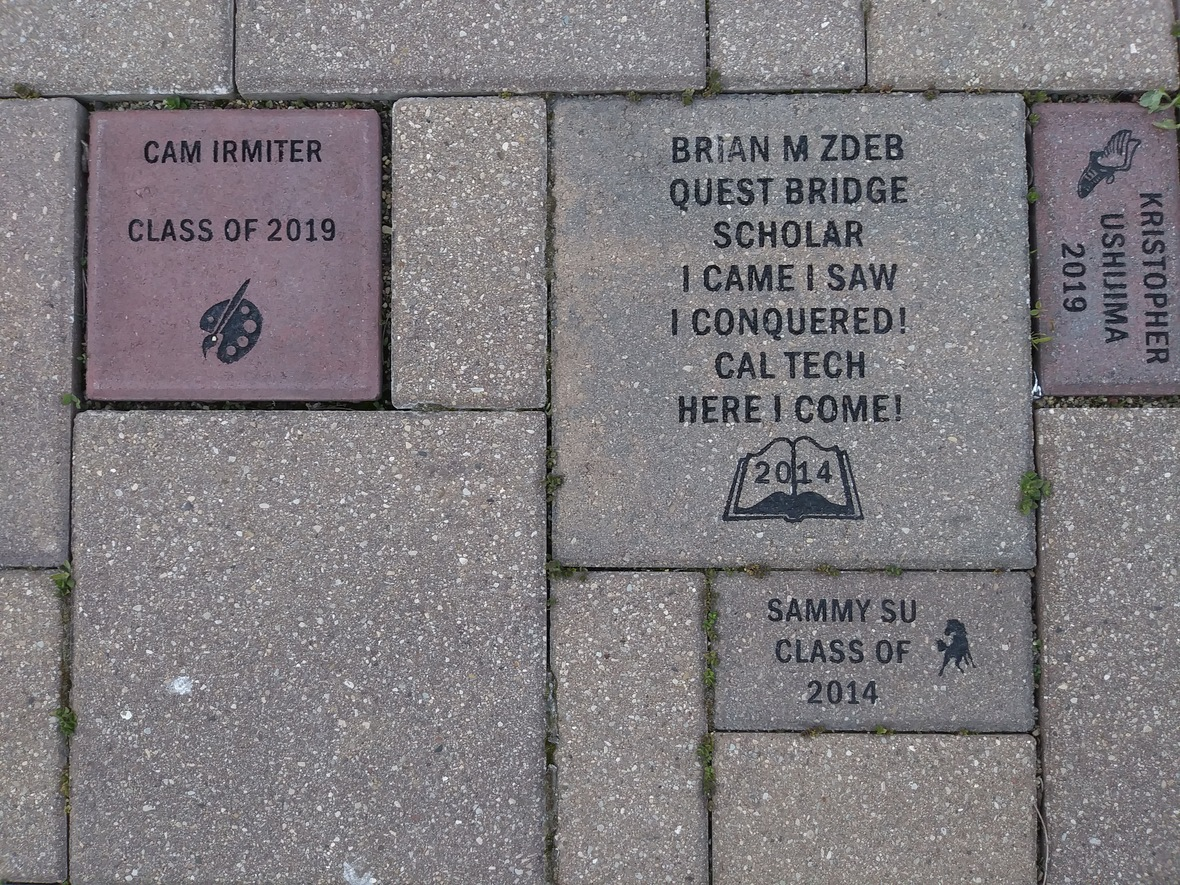Struggling to find one last gift for your child this year? Consider buying a custom NCP Brick. Your brick, engraved with a personal message, will become a permanent part of the Northside College Prep Celestial Garden Walkway.

Your brick may be purchased in three sizes:

▪ 4" x 8" = \$50
▪ 8" x 8" = \$100
▪ 12" x 12" = \$150

Friends of Northside will provide a gift certificate acknowledging the purchase that can be given during the holiday season. Janet Jasmer will assist with the process and can answer any questions at jjasmer@atproperties.com.

 table div table+table+table+table+table+table+table+table+table+table+table+table+table+table+table+table+table+table+table+table+table+table+table div table{width:100%;padding:0}table div table+table+table+table+table+table+table+table+table+table+table+table+table+table+table+table+table+table+table+table+table+table+table div table img{width:96.23%;padding:0;float:none}table div table+table+table+table+table+table+table+table+table+table+table+table+table+table+table+table+table+table+table+table+table+table+table div table td{width:100%;padding:0 1.88% 18px}/* styles */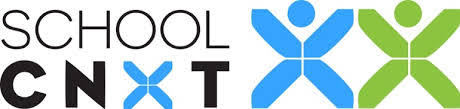# Any Questions About NCP Communications Sent Via CNXT?

If you are having problems with SchoolCNXT, please contact the main office from 7:30 am - 3:15 pm at 773.534.3954, or you can email the NCP school clerk, Mary Grasz, at mtgrasz@cpu.edu.

NCP uses SchoolCNXT to communicate important information to parents and the school community. All families should have received a text message and/or email communication about signing up. If you did not receive that communication or need access to your student's account, you can gain access by using this tool on Northside's website.

 table div table+table+table+table+table+table+table+table+table+table+table+table+table+table+table+table+table+table+table+table+table+table+table+table+table+table div table{width:100%;padding:0}table div table+table+table+table+table+table+table+table+table+table+table+table+table+table+table+table+table+table+table+table+table+table+table+table+table+table div table img{width:96.23%;padding:0;float:none}table div table+table+table+table+table+table+table+table+table+table+table+table+table+table+table+table+table+table+table+table+table+table+table+table+table+table div table td{width:100%;padding:0 1.88% 18px}/* styles */# CPS Scholarship Notifications and College Events Around the City

For more details about upcoming events and to access the most recent updates, (click HERE) to view the College Calendar on the Northside Prep Counseling Website. Also, for additional scholarship opportunities visit the Northside College Prep Counseling page (click HERE).

Saturday, January 9, 2021
App Closes: Ron Brown Scholar Program

Thursday, January 14, 2021
App Closes: Department of the Air Force - Type 1 Scholarship
App Closes: Department of the Air Force - Type 2 Scholarship
App Closes: Department of the Air Force - Type 7 Scholarship

Friday, January 15, 2021
App Closes: Carson Scholars Fund
App Closes: Finance Academy Scholarship (Winter)
App Closes: The Diversity Advancement Scholarship

 table div table+table+table+table+table+table+table+table+table+table+table+table+table+table+table+table+table+table+table+table+table+table+table+table+table+table+table+table div table{width:100%;padding:0}table div table+table+table+table+table+table+table+table+table+table+table+table+table+table+table+table+table+table+table+table+table+table+table+table+table+table+table+table div table img{width:96.23%;padding:0;float:none}table div table+table+table+table+table+table+table+table+table+table+table+table+table+table+table+table+table+table+table+table+table+table+table+table+table+table+table+table div table td{width:100%;padding:0 1.88% 18px}/* styles */# College Representative Visits

The NCP Counseling Department is offering virtual postsecondary visits for Northside Prep students and parents. We are offering virtual postsecondary visits to ensure all families have the opportunity to explore various postsecondary pathways, engage with the postsecondary representatives, and obtain answers to pertinent questions or concerns. Virtual postsecondary visits are offered after school from 3:15 pm - 4:00 pm and during Flex Blocks 1-4 on Wednesdays.

To date, college, military, and GAP Year representatives are scheduled to connect with NCP students virtually, and the list is growing every day. Click HERE to view the list of upcoming and past postsecondary visits. Also, check out the messages from the admissions representatives and the additional resources they shared during their visits.

*Please note, all virtual visits will be conducted via CPS Google Meets and no email addresses outside CPS will be permitted into the virtual postsecondary visits. To protect the privacy and safety of students, parents will only be permitted to access virtual visits if their respective student is present and the student will be required to log in via their CPS Gmail account. All postsecondary visits will be managed and monitored by an NCP staff member.

 table div table+table+table+table+table+table+table+table+table+table+table+table+table+table+table+table+table+table+table+table+table+table+table+table+table+table+table+table+table+table div table{width:100%;padding:0}table div table+table+table+table+table+table+table+table+table+table+table+table+table+table+table+table+table+table+table+table+table+table+table+table+table+table+table+table+table+table div table img{width:96.23%;padding:0;float:none}table div table+table+table+table+table+table+table+table+table+table+table+table+table+table+table+table+table+table+table+table+table+table+table+table+table+table+table+table+table+table div table td{width:100%;padding:0 1.88% 18px}/* styles */# Photo Submissions Requested for NCP Stampede!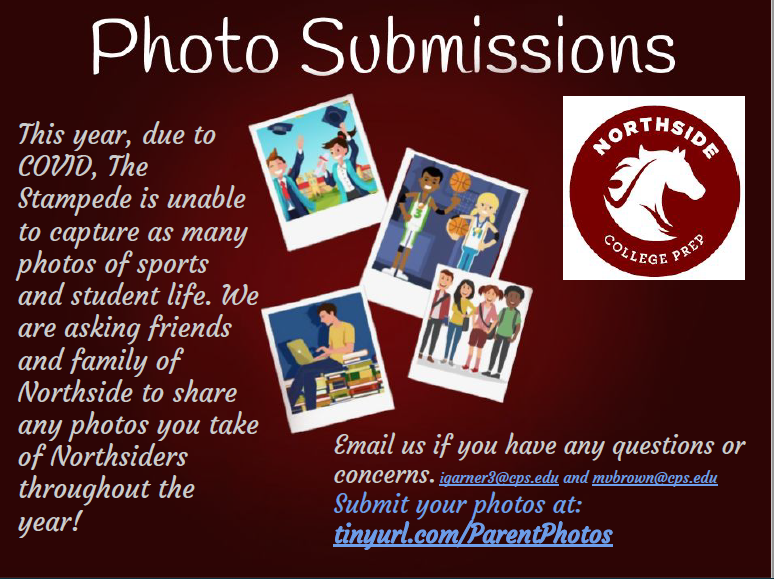table div table+table+table+table+table+table+table+table+table+table+table+table+table+table+table+table+table+table+table+table+table+table+table+table+table+table+table+table+table+table+table+table+table div table{width:100%;padding:0}table div table+table+table+table+table+table+table+table+table+table+table+table+table+table+table+table+table+table+table+table+table+table+table+table+table+table+table+table+table+table+table+table+table div table img{width:96.23%;padding:0;float:none}table div table+table+table+table+table+table+table+table+table+table+table+table+table+table+table+table+table+table+table+table+table+table+table+table+table+table+table+table+table+table+table+table+table div table td{width:100%;padding:0 1.88% 18px}/* styles */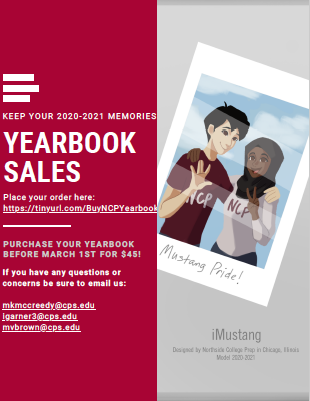# Order a Yearbook Today!

Copies are \$45 until March 1 and \$55 afterwards.

 table div table+table+table+table+table+table+table+table+table+table+table+table+table+table+table+table+table+table+table+table+table+table+table+table+table+table+table+table+table+table+table+table+table+table+table+table div table{width:100%;padding:0}table div table+table+table+table+table+table+table+table+table+table+table+table+table+table+table+table+table+table+table+table+table+table+table+table+table+table+table+table+table+table+table+table+table+table+table+table div table img{width:96.23%;padding:0;float:none}table div table+table+table+table+table+table+table+table+table+table+table+table+table+table+table+table+table+table+table+table+table+table+table+table+table+table+table+table+table+table+table+table+table+table+table+table div table td{width:100%;padding:0 1.88% 18px}/* styles */# Do you have news or team results to share with the Northside parent community?

 table div table+table+table+table+table+table+table+table+table+table+table+table+table+table+table+table+table+table+table+table+table+table+table+table+table+table+table+table+table+table+table+table+table+table+table+table+table+table+table div table{width:100%;padding:0}table div table+table+table+table+table+table+table+table+table+table+table+table+table+table+table+table+table+table+table+table+table+table+table+table+table+table+table+table+table+table+table+table+table+table+table+table+table+table+table div table img{width:96.23%;padding:0;float:none}table div table+table+table+table+table+table+table+table+table+table+table+table+table+table+table+table+table+table+table+table+table+table+table+table+table+table+table+table+table+table+table+table+table+table+table+table+table+table+table div table td{width:100%;padding:0 1.88% 18px}/* styles */# Support Northside - Shop AmazonSmile

AmazonSmile is a simple and automatic way to support Northside every time you shop.

Please note that our AmazonSmile name is Friends of "North" "Side" (North Side - two words at AmazonSmile).

 table div table+table+table+table+table+table+table+table+table+table+table+table+table+table+table+table+table+table+table+table+table+table+table+table+table+table+table+table+table+table+table+table+table+table+table+table+table+table+table+table+table div table{width:100%;padding:0}table div table+table+table+table+table+table+table+table+table+table+table+table+table+table+table+table+table+table+table+table+table+table+table+table+table+table+table+table+table+table+table+table+table+table+table+table+table+table+table+table+table div table img{width:96.23%;padding:0;float:none}table div table+table+table+table+table+table+table+table+table+table+table+table+table+table+table+table+table+table+table+table+table+table+table+table+table+table+table+table+table+table+table+table+table+table+table+table+table+table+table+table+table div table td{width:100%;padding:0 1.88% 18px}/* styles */# Follow Us on Social Media

 table div table+table+table+table+table+table+table+table+table+table+table+table+table+table+table+table+table+table+table+table+table+table+table+table+table+table+table+table+table+table+table+table+table+table+table+table+table+table+table+table+table+table+table div table{width:100%;padding:0}table div table+table+table+table+table+table+table+table+table+table+table+table+table+table+table+table+table+table+table+table+table+table+table+table+table+table+table+table+table+table+table+table+table+table+table+table+table+table+table+table+table+table+table div table img{width:96.23%;padding:0;float:none}table div table+table+table+table+table+table+table+table+table+table+table+table+table+table+table+table+table+table+table+table+table+table+table+table+table+table+table+table+table+table+table+table+table+table+table+table+table+table+table+table+table+table+table div table td{width:100%;padding:0 1.88% 18px}/* styles */table div table+table+table+table+table+table+table+table+table+table+table+table+table+table+table+table+table+table+table+table+table+table+table+table+table+table+table+table+table+table+table+table+table+table+table+table+table+table+table+table+table+table+table+table+table div table{width:100%;padding:0}table div table+table+table+table+table+table+table+table+table+table+table+table+table+table+table+table+table+table+table+table+table+table+table+table+table+table+table+table+table+table+table+table+table+table+table+table+table+table+table+table+table+table+table+table+table div table img{width:96.23%;padding:0;float:none}table div table+table+table+table+table+table+table+table+table+table+table+table+table+table+table+table+table+table+table+table+table+table+table+table+table+table+table+table+table+table+table+table+table+table+table+table+table+table+table+table+table+table+table+table+table div table td{width:100%;padding:0 1.88% 18px}/* styles */table div table+table+table+table+table+table+table+table+table+table+table+table+table+table+table+table+table+table+table+table+table+table+table+table+table+table+table+table+table+table+table+table+table+table+table+table+table+table+table+table+table+table+table+table+table+table+table+table div table{width:100%;padding:0}table div table+table+table+table+table+table+table+table+table+table+table+table+table+table+table+table+table+table+table+table+table+table+table+table+table+table+table+table+table+table+table+table+table+table+table+table+table+table+table+table+table+table+table+table+table+table+table+table div table img{width:96.23%;padding:0;float:none}table div table+table+table+table+table+table+table+table+table+table+table+table+table+table+table+table+table+table+table+table+table+table+table+table+table+table+table+table+table+table+table+table+table+table+table+table+table+table+table+table+table+table+table+table+table+table+table+table div table td{width:100%;padding:0 1.88% 18px}/* styles */